# Bregman distance

Given a convex closed set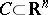with non-empty interior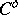and a Bregman functionwith zone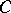, the Bregman distance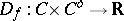is defined as: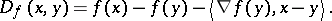Bregman distances were introduced in [a1]. For several examples of Bregman distances for relevant sets, see Bregman function. It follows easily from the properties of Bregman functions that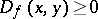for all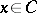and all, that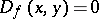if and only if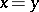and that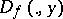is a convex function (cf. also convex function (of a real variable)) for all. In general,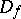does not satisfy the triangle inequality, it is not symmetric (i.e. it is not true thatfor all,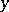) and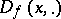is not convex. If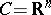and eitheris symmetric oris convex for all, thenis a quadratic function andis the square of an elliptic norm. A basic property of Bregman distances, which follows easily from the definition, is the following: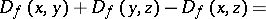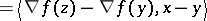for all, and all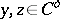. Given a closed convex set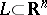such that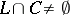, the Bregman projection onto,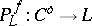, is defined asThe properties of Bregman distances ensure existence and uniqueness of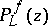for all. Given closed convex sets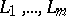such thatfor alland all(such sets are said to be zones consistent with), it is interesting to consider a sequence of successive Bregman projections onto the convex sets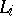, i.e. the sequence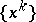withand iterative formula given by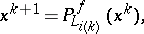whereis the index of the convex set used in theth iteration (for instance cyclically, i.e.). This algorithm, called Bregman's method, converges to a point in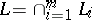ifis non-empty (see [a1]). It has been proved in [a1] that if all the setsare hyperplanes, then the limit of the sequenceis also the unique solution of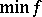, subject to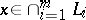. This property also holds for an underrelaxed version of the method, of the type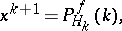where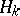is a hyperplane parallel to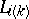and lying betweenand(see [a3]). Under suitable modifications in the definition of the hyperplane, the method has been extended to the case of minimization ofsubject to linear inequalities and linear interval constraints (see [a2], [a5]). The entropy maximization method known as MART (multiplicative algebraic reconstruction technique, see [a4]) is a particular case of Bregman's method with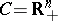(the non-negative orthant of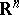) and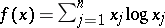, under a specific underrelaxation strategy.

Bregman distances have also been used to generate generalized proximal point methods for convex optimization and variational inequalities (cf. Proximal point methods in mathematical programming).